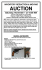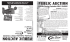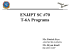# A Readable Introduction to Real Mathematics

## Transcription

A Readable Introduction to Real Mathematics
```Selected solutions to problems in the book
A Readable Introduction to Real Mathematics
D. Rosenthal, D. Rosenthal, P. Rosenthal
Chapter 1: Introduction to the Natural Numbers
1. Show that the following are composite numbers:
(c) 20,101,116
Answer: 20,101,116 is divisible by 2.
2. Which of the following are prime numbers?
(c) 537
Answer: Since 537 is divisible by 3, it is not prime.
6. Find a prime number p such that the number (2 · 3 · 5 · 7 · · · p) + 1 is not prime.
Answer: The smallest such prime is p = 13. Note that 2 · 3 · · · 13 = 30030 and
30031 = 59 · 509.
7. Suppose that p, p + 2, and p + 4 are prime numbers. Prove that p = 3.
[Hint: Why can’t p be 5 or 7?]
Answer: If p = 3, then p, p + 2 = 5 and p + 4 = 7 are all prime. Conversely, suppose
p 6= 3. Then, since p is prime, p is not divisible by 3. Thus, p leaves a remainder of 1 or
2 when divided by 3. If p leaves a remainder of 1 when divided by 3, then p = 3m + 1
for some nonnegative integer m, so p+2 = 3m+3 is divisible by 3. Since p+2 is greater
than 3, this contradicts the fact that p + 2 is prime. If p leaves a remainder of 2 when
divided by 3, then p = 3m + 2 for some nonnegative integer m, and so p + 4 = 3m + 6
2
is divisible by 3, which contradicts the fact that p + 4 is prime. Therefore, for p, p + 2
and p + 4 to all be prime, p must equal 3.
8. Prove that, for every natural number n > 2, there is a prime number between n and n!.
(Recall that n! is defined to be n(n − 1)(n − 2) · · · 2 · 1.)
[Hint: There is a prime number that divides n! − 1.]
Note that this gives an alternate proof that there are infinitely many prime numbers.
Answer: Let n be a natural number greater than 2. Let p be any prime number that
divides n! − 1. Since p divides n! − 1, p does not divide n!. It follows that p is not any
natural number less than or equal to n, and so p is a natural number greater than n.
Also, p is less than or equal to n! − 1, since p divides n! − 1. It follows that n < p < n!,
so there is a prime between n and n!.
9. Prove that, for every natural number n, there are n consecutive composite numbers.
[Hint: (n + 1)! + 2 is a composite number.]
Answer: Let n be any natural number. For any natural number k ≤ n + 1, (n + 1)! is
a multiple of k. Then (n + 1)! + k is k more than a multiple of k, so (n + 1)! + k is a
multiple of k as well. Thus (n + 1)! + 2, (n + 1)! + 3, (n + 1)! + 4, . . . , (n + 1)! + n + 1
are n consecutive numbers all of which are composite.
10. Show that a natural number has an odd number of different factors if and only if it is a
perfect square (i.e., it is the square of another natural number).
Answer: Let m be a natural number whose distinct factors are a1 , . . . , an . For each ai ,
there is an aj such that ai · aj = m. So each factor can be paired with its complement,
and if m is not a perfect square then each ai is paired with a different aj , so there
are an even number of factors. If m is a perfect square, then there is an ak such that
a2k = m. All the other ai can be paired with their complements, but as ak only appears
once in the list of factors, it follows that there are an odd number of different factors.
3
Chapter 2: Mathematical Induction
2. Prove, using induction, that for every natural number n:
1
1
1
n
+
+ ··· +
=
1·2 2·3
n · (n + 1)
n+1
Answer: This is true when n = 1, since
1
1·2
=
1
1+1 .
Suppose it is true for n = k, i.e.:
1
1
1
k
+
+ ··· +
=
1·2 2·3
k · (k + 1)
k+1
Then:
1
1
1
1
+
+ ··· +
+
1·2 2·3
k · (k + 1) (k + 1) · (k + 2)
=
k
1
+
k + 1 (k + 1)(k + 2)
=
k(k + 2) + 1
(k + 1)(k + 2)
=
k 2 + 2k + 1
(k + 1)(k + 2)
=
=
(k + 1)(k + 1)
(k + 1)(k + 2)
k+1
k+2
Therefore, the formula holds for n = k + 1, and it follows by mathematical induction
that the formula is true for all natural numbers n.
7. Prove by mathematical induction that 3 divides n3 + 2n, for every natural number n.
Answer: For the case n = 1, n3 + 2n = 1 + 2 = 3, so the statement holds in this
case. Suppose it holds for the natural number k. Then (k + 1)3 + 2(k + 1) = (k 3 +
3k 2 + 3k + 1) + (2k + 2) = k 3 + 2k + 3k + 3k 2 + 3 = (k 3 + 2k) + 3(k + k 2 + 1). Since
k 3 + 2k is divisible by 3, by the inductive hypothesis, and 3(k + k 2 + 1) is divisible by
3, (k + 1)3 + 2(k + 1) is divisible by 3, and the result follows by induction.
4
8. Show that 3n > n2 for every natural number n.
Answer: For n = 1, 3n = 3 while n2 = 1, so the inequality holds in this case. For
n = 2, 3n = 9 > 4 = n2 , so it is true in this case as well. Suppose it is true for a natural
number k ≥ 2, that is, 3k > k 2 . Then 3k+1 = 3 · 3k > 3k 2 , while (k + 1)2 = k 2 + 2k + 1.
What remains is to show that 3k 2 > k 2 + 2k + 1 or, equivalently, 2k 2 > 2k + 1. This
is equivalent to 2k 2 − 2k > 1, but 2k 2 − 2k = 2k(k − 1), which is clearly greater than
1 for k ≥ 2.
12. Prove the Well-Ordering Principle using the Principle of Complete Mathematical Induction.
Answer: It must be shown that the only set without a smallest element is the empty
set. Let T be a set of natural numbers without a smallest element. Then 1 is not in
T , or else 1 would be the smallest element. Suppose the numbers 1 through k are not
in T . Then k + 1 is also not in T , or else k + 1 would be the smallest element in T .
So if S is the set of natural numbers that are not in T , then 1 is in S and k + 1 is in S
whenever the numbers 1 through k are in S. It follows from the Principle of Complete
Mathematical Induction that S is the set of all natural numbers. Therefore, there are
no natural numbers in T ; that is, T is the empty set.
n
14. Define the nth Fermat number, Fn , by Fn = 22 + 1 for n = 0, 1, 2, 3, . . . . The first few
Fermat numbers are F0 = 3, F1 = 5, F2 = 17, F3 = 257.
(a) Prove by induction that F0 · F1 · · · Fn−1 + 2 = Fn , for n ≥ 1.
(b) Use the formula in part (a) to prove that there are an infinite number of primes, by
showing that no two Fermat numbers have any prime factors in common.
[Hint: For each Fn , let pn be a prime divisor of Fn and show that pn1 6= pn2 if n1 6= n2 .]
Answer: (a) For n = 1 the left hand side is 3 + 2 while the right hand side is 5, so
the statement holds. Suppose the statement is true for a natural number m. Then
F0 · F1 · · · Fm−1 + 2 = Fm , so:
5
F0 · F1 · · · Fm−1 = Fm − 2
m
= 22 + 1 − 2
m
= 22 − 1
It follows that:
m
F0 · F1 · · · Fm−1 · Fm = (22 − 1) · Fm
m
m
= (22 − 1)(22 + 1)
m+1
= 22
−1
= Fm+1 − 2
Therefore:
F0 · F1 · · · Fm−1 · Fm + 2 = Fm+1
and the statement holds for m + 1.
(b) Let n and m be two different natural numbers, and suppose that Fn and Fm share
the prime factor p. One of n and m is greater than the other; assume n > m. Then,
by the formula from part (a):
Fn = F0 · F1 · · · Fn−1 + 2
Since Fm is one of the Fi on the right hand side of this equation, p divides the product
on the right hand side. Since p also divides Fn , it follows that p divides 2, and hence
p = 2. On the other hand, every Fermat number is odd, so 2 is not a factor of any
Fermat number. Since no two Fermat numbers have a common prime factor, this
provides another proof that there are an infinite number of primes.
15. The sequence of Fibonacci numbers is defined as follows: x1 = 1, x2 = 1, and, for n > 2,
xn = xn−1 + xn−2 . Prove that:
1
xn = √
5
"
√ !n
1+ 5
−
2
√ !n #
1− 5
2
for every natural number n.
[Hint: Use the fact that x =
√
1+ 5
2
and x =
√
1− 5
2
both satisfy 1 + x = x2 .]
6
Answer: To verify the hint, note that
√ 2
√
√
√
6−2 5
1− 5
3− 5
1− 5
=
=
=
1
+
2
4
2
2 .
√ 2
1+ 5
2
=
√
6+2 5
4
=
√
3+ 5
2
= 1+
√
1+ 5
2 ,
and
For the base case of the induction, x1 = 1 by definition and:
"
√ !#
√ !
√ !
1− 5
1
1+ 5
2 5
1
√
−
=√
=1
2
2
2
5
5
Suppose the formula holds for all natural numbers less than or equal to k. Then:
xk+1 = xk + xk−1

√ !k
√ !k
√ !k−1
√ !k−1
1+ 5
1
1− 5
1+ 5
1− 5

√ 
−
+
−
2
2
2
2
5


√ !
√ !k−1
√ !
√ !k−1
1+ 5
1− 5
1− 5 
1+ 5
1
√ 
1+
−
1+
2
2
2
2
5

=
=
and, by the hint, this equals:

√ !k−1
√ !2
1  1+ 5
1+ 5
√
−
2
2
5
√ !k−1
1− 5
2

√ !2
1− 5 
2
Since this is equal to the right hand side of the formula for n = k + 1, the result follows
by induction.
Chapter 3: Modular Arithmetic
1. Find a solution x to each of the following congruences. (“Solution” means integer solution.)
(a) 2x ≡ 7 (mod 11)
Answer: If there is a solution, there would have to be a solution within the set
{0, 1, . . . , 10}. Trying each of those numbers gives the solution x = 9.
7
2. For each of the following congruences, either find a solution or prove that no solution exists.
(a) 39x ≡ 13 (mod 5)
(b) 95x ≡ 13 (mod 5)
(e) 4x3 + 2x ≡ 7 (mod 5)
Answer: (a) Note that if there is a solution to a congruence modulo 5, there would have
to be a solution among the set {0, 1, 2, 3, 4}, since every integer is congruent to one of
those numbers modulo 5. Since 39 ≡ 4 (mod 5), and 13 ≡ 3 (mod 5), the equation
is equivalent to 4x ≡ 3 (mod 5). Trying each number from {0, 1, 2, 3, 4} yields the
solution x = 2.
(b) No solution exists. None of the numbers from {0, 1, 2, 3, 4} satisfy the congruence.
Another solution is as follows. For any x, 95x ≡ 0 (mod 5), because 95 ≡ 0 (mod 5).
Since 13 ≡ 3 (mod 5), there is no solution.
(e) No solution exists. None of the numbers from {0, 1, 2, 3, 4} satisfy the congruence.
3. Find the remainder when:
(c) 243101 is divided by 8.
(g) 5! · 181 − 866 · 332 is divided by 6.
Answer: (c) First note that 243 ≡ 3 (mod 8), so 2432 ≡ 32 ≡ 1 (mod 8). Then
243100 ≡ 1 (mod 8), so 243101 ≡ 243 ≡ 3 (mod 8). Therefore the remainder is 3.
(g) The remainder is 2. To see this, note that 866 ≡ 2 (mod 6) and 332 ≡ 2 (mod 6),
so −866·332 ≡ −4 ≡ 2 (mod 6). Since 5! = 20·6, 5! ≡ 0 (mod 6), therefore 5!·181 ≡ 0
(mod 6). Then, 5! · 181 − 866 · 332 ≡ 2 (mod 6).
7. Suppose that 722 is written out in the ordinary way. What is its last digit?
Answer: Note that the last digit (i.e., the units’ digit) of every natural number is
the remainder that the natural number leaves upon division by 10. In the present
case, since 72 ≡ 9 (mod 10), it follows that 73 ≡ 63 ≡ 3 (mod 10) and 74 ≡ 21 ≡ 1
8
(mod 10). Then 720 ≡ 1 and so 722 ≡ 72 ≡ 9 (mod 10). Therefore, the remainder
upon division by 10 is 9, hence, the last digit is 9.
13. Find the units’ digit of 274936782 .
Answer: This is equivalent to finding the remainder that 274936782 leaves upon division
by 10. To find this, first note that 27493 ≡ 3 (mod 10), so 274932 ≡ 9 ≡ −1 (mod 10),
and 274934 ≡ 1 (mod 10). It follows that 274936780 ≡ 1 (mod 10), since 6780 is a
multiple of 4. Therefore, 274936782 ≡ (274936780 )(274932 ) ≡ 9 · 1 (mod 10) and the
units’ digit is 9.
15. Prove that, for every pair of natural numbers m and n, m2 is congruent to n2 modulo
(m + n).
Answer: Since m2 − n2 = (m − n)(m + n), it follows that m2 − n2 is divisible by m + n.
Thus, m2 ≡ n2 (mod m + n).
17. Prove that 7 divides 82n+1 + 62n+1 , for every natural number n.
Answer: Note that 8 ≡ 1 (mod 7), so 82n+1 ≡ 1 (mod 7). Also, 6 ≡ −1 (mod 7), so
62n+1 ≡ (−1)2n+1 ≡ −1 (mod 7). Thus, 82n+1 + 62n+1 ≡ 1 + (−1) ≡ 0 (mod 7).
Alternatively, this can be shown by induction. Since 83 +63 = 512+216 = 728 = 7·104,
the formula holds for n = 1. Now assume that 82k+1 + 62k+1 is divisible by 7. Then
82(k+1)+1 + 62(k+1)+1 = 82 (82k+1 + 62k+1 ) − 28(62k+1 ), which is congruent to (0 + 0)
modulo 7. Therefore the statement is true for k + 1.
18. Prove that a natural number that is congruent to 2 modulo 3 has a prime factor that is
congruent to 2 modulo 3.
Answer: Let m be an integer greater than 1 such that m ≡ 2 (mod 3). If m is prime,
then m itself is the prime factor congruent to 2 modulo 3. If m is not prime, then m
9
can be factored into primes q1 · q2 · · · qn . If all of the prime factors were congruent to
1 mod 3, then m would be congruent to 1n ≡ 1 mod 3, and if any prime factor was
congruent to 0 mod 3 then m would be congruent to 0 as well. Thus, there must be
at least one prime factor of m that is not congruent to 1 mod 3 and that prime factor
cannot be congruent to 0 mod 3. Since every number is congruent to one of 0, 1, 2
modulo 3, it follows that at least one prime factor must be congruent to 2 mod 3.
22. Show that there do not exist natural numbers x and y such that x2 + y 2 = 4003.
[Hint: Begin by determining which of the numbers {0, 1, 2, 3} can be congruent to x2
(mod 4).]
Answer: Suppose there were such x and y. Then x2 + y 2 ≡ 4003 ≡ 3 (mod 4).
However, since 02 ≡ 0, 12 ≡ 1, 22 ≡ 0 and 32 ≡ 1 modulo 4, the only possible numbers
in {0, 1, 2, 3} that x2 + y 2 could be congruent to modulo 4 are 0, 1, 2.
24. Prove that there are an infinite number of primes of the form 4k + 3 with k a natural
number.
[Hint: If p1 , p2 , . . . , pn are n such primes, show that (4 · p1 · p2 · · · pn ) − 1 has at least one
prime divisor of the given form.]
Answer: We first prove the equivalent statement for primes of the form 4k + 3 where
k is any nonnegative integer (thus including the case k = 0). Since 4 · 1 + 3 = 7, and 7
is prime, there is at least one prime of that form. Let p1 , . . . , pn be primes of the given
form. Factor 4(p1 · · · pn ) − 1 into primes q1 · · · qj . For each pi , 4(p1 · · · pn ) − 1 is one
less than a multiple of pi , so there are no pi and qt with pi = qt . Also 4(p1 · · · pn ) − 1 ≡
−1 ≡ 3 (mod 4), so q1 · · · qj ≡ 3 (mod 4). If none of the qt ’s were congruent to 3
modulo 4, then each would be congruent to 0, 1, or 2 modulo 4, and their product
would be congruent to 0, 1, or 2 modulo 4. So one of the qt ’s must be congruent to 3
modulo 4, and so qt = 4k + 3 for some integer k. Therefore, given any n primes of the
given form, it is always possible to find another one. Thus, there are infinitely many
10
of them. In particular, there are infinitely many where k is a natural number (since
the case k = 0 only gives one prime, 3).
27. Show that, if x, y and z are integers such that x2 + y 2 = z 2 , then at least one of {x, y, z}
is divisible by 2, at least one of {x, y, z} is divisible by 3, and at least one of {x, y, z} is
divisible by 5.
Answer: Suppose that none are divisible by 2. Then each of x, y and z is congruent to
1 modulo 2, so x2 ≡ y 2 ≡ z 2 ≡ 1 (mod 2). However x2 + y 2 ≡ z 2 (mod 2), giving the
equation 1 + 1 ≡ 1 (mod 2), a contradiction. Therefore at least one is divisible by 2.
Suppose none are divisible by 3. Then each is congruent to 1 or 2 modulo 3. Since
22 ≡ 1 ≡ 12 (mod 3), it follows that each of x2 , y 2 and z 2 is congruent to 1 modulo 3,
and the equation x2 + y 2 ≡ z 2 (mod 3) gives 1 + 1 ≡ 1 (mod 3), a contradiction.
Finally, suppose none are divisible by 5. Then each is congruent to 1, 2, 3, or 4 modulo
5. Since 12 ≡ 1 (mod 5), 22 ≡ 4 (mod 5), 32 ≡ 4 (mod 5) and 42 ≡ 1 (mod 5), it
follows that each of x2 , y 2 and z 2 is congruent to 1 or 4 modulo 5. Substituting these
into the equation x2 + y 2 ≡ z 2 (mod 5) shows that this is not possible, as 1 + 1 ≡ 2
(mod 5), 1 + 4 ≡ 4 + 1 ≡ 0 (mod 5), and 4 + 4 ≡ 3 (mod 5).
```

### armedforces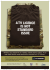### Homework 3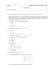### Discrete Math, Spring 2013 - Some Sample Problems### Avaya Part Number: 700415656 IP Phone 1616/32B MOD DESIG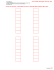### Semester I Examinations 2013-14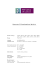### Untitled - Unfilodi### Modular Math - Walton High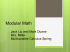### AUCTION INVENTORY REDUCTION &amp; MOVING Saturday, November 1 @ 10:00 AM